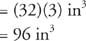## Right Prisms

In certain prisms, the lateral faces are each perpendicular to the plane of the base (or bases if there is more than one). These are known as a group as right prisms.

## Lateral area of a right prism

The lateral area of a right prism is the sum of the areas of all the lateral faces.

Theorem 87: The lateral area, LA, of a right prism of altitude h and perimeter p is given by the following equation.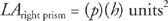Example 1: Find the lateral area of the right hexagonal prism, shown in Figure 1.Figure 1 A right hexagonal prism.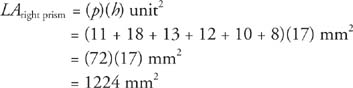The total area of a right prism is the sum of the lateral area and the areas of the two bases. Because the bases are congruent, their areas are equal.

Theorem 88: The total area, TA, of a right prism with lateral area LA and a base area B is given by the following equation.Example 2: Find the total area of the triangular prism, shown in Figure 2.Figure 2 A (right) triangular prism.

The base of this prism is a right triangle with legs of 3 ft and 4 ft (Figure 3).Figure 3 The base of the triangular prism from Figure 2.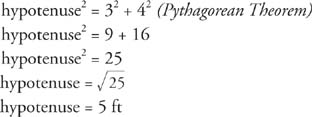The perimeter of the base is (3 + 4 + 5) ft, or 12 ft.

Because the triangle is a right triangle, its legs can be used as base and height of the triangle.The altitude of the prism is given as 2 ft. Therefore,## Interior space of a solid

Lateral area and total area are measurements of the surface of a solid. The interior space of a solid can also be measured.

cube is a square right prism whose lateral edges are the same length as a side of the base; see Figure 4.Figure 4 A cube.

The volume of a solid is the number of cubes with unit edge necessary to entirely fill the interior of the solid. In Figure 5, the right rectangular prism measures 3 inches by 4 inches by 5 inches.Figure 5 Volume of a right rectangular prism.

This prism can be filled with cubes 1 inch on each side, which is called a cubic inch. The top layer has 12 such cubes. Because the prism has 5 such layers, it takes 60 of these cubes to fill this solid. Thus, the volume of this prism is 60 cubic inches.

Theorem 89: The volume, V, of a right prism with a base area B and an altitude h is given by the following equation.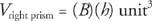Example 3: Figure 6 is an isosceles trapezoidal right prism. Find (a) LA (b) TA and (c) V.Figure 6 An isosceles trapezoidal right prism.

• (a)

LA right prism = ( p)( h) units2

Note: The h refers to the altitude of the prism, not the height of the trapezoid.)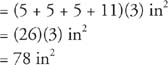•• (c)

V right prism = ( B)( h) units3

Note: The h refers to the altitude of the prism, not the height of the trapezoid.)